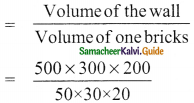Students can download Maths Chapter 7 Mensuration Ex 7.4 Questions and Answers, Notes, Samacheer Kalvi 9th Maths Guide Pdf helps you to revise the complete Tamilnadu State Board New Syllabus, helps students complete homework assignments and to score high marks in board exams.

## Tamilnadu Samacheer Kalvi 9th Maths Solutions Chapter 7 Mensuration Ex 7.4

Question 1.
The semi-perimeter of a triangle having sides 15 cm, 20 cm and 25 cm is ……..
(a) 60 cm
(b) 45 cm
(c) 30 cm
(d) 15 cm
Solution:
(c) 30 cm
Hint:
l = 15 cm, b = 20 cm, h = 25 cm
Semi-perimeter = $$\frac{a+b+c}{2}$$
= $$\frac{15+20+25}{2}$$
= 30 cmQuestion 2.
If the sides of a triangle are 3 cm, 4 cm and 5 cm, then the area is ………
(a) 3 cm²
(b) 6 cm²
(c) 9 cm²
(d) 12 cm²
Solution:
(b) 6 cm²
Hint:
a- 3 cm, b = 4 cm, c = 5 cm
s = $$\frac{a+b+c}{2}$$
= $$\frac{3+4+5}{2}$$
= 6 cm
Area of the triangle = $$\sqrt{s(s-a)(s-b)(s-c)}$$
= $$\sqrt{6×3×2×1}$$
= $$\sqrt{36}$$
= 6 cm²Question 3.
The perimeter of an equilateral triangle is 30 cm. The area is ……..
(a) 10 √3 cm²
(b) 12 √3 cm²
(c) 15 √3 cm²
(d) 25 √3 cm²
Solution:
(d) 25 √3 cm²
Hint:
Perimeter of an equilateral triangle = 30 cm
3a = 30 cm
a = $$\frac{30}{3}$$
= 10 cm
Area of an equilateral triangle = $$\frac{√3}{4}$$ a² sq.units
= $$\frac{√3}{4}$$ × 10 × 10
= 25 √3 cm²Question 4.
The lateral surface area of a cube of side 12 cm is ……..
(a) 144 cm²
(b) 196 cm²
(c) 576 cm²
(d) 664 cm²
Solution:
(c) 576 cm²
Hint:
Side of a cube (a) = 12 cm
L.S.A. of a cube = 4a² sq.units
= 4 × 12 × 12 cm²
= 576 cm²Question 5.
If the lateral surface area of a cube is 600 cm², then the total surface area is ………
(a) 150 cm²
(b) 400 cm²
(c) 900 cm²
(d) 1350 cm²
Solution:
(c) 900 cm²
Hint:
L.S.A. of a cube = 600 cm²
4a² = 600
a² = $$\frac{600}{4}$$
= 150
Total surface area of a cube = 6a² sq.units
= 6 × 150 cm²
= 900 cm²Question 6.
The total surface area of a cuboid with dimension 10 cm × 6 cm × 5 cm is ………
(a) 280 cm²
(b) 300 cm²
(c) 360 cm²
(d) 600 cm²
Solution:
(a) 280 cm²
Hint:
T.S.A. of a cuboid = 2(lb + bh + lh) sq.units
= 2(10 × 6 + 6 × 5 + 10 × 5) cm²
= 2(60 + 30 + 50) cm²
= 2 × 140 cm²
= 280 cm²

Question 7.
If the ratio of the sides of two cubes are 2 : 3, then ratio of their surface areas will be ………
(a) 4 : 6
(b) 4 : 9
(c) 6 : 9
(d) 16 : 36
Solution:
(b) 4 : 9
Hint:
Ratio of the surface area of cubes = 4a12 : 4a22
= a12 : a22
= 4² : 9²
= 4 : 9Question 8.
The volume of a cuboid is 660 cm and the area of the base is 33 cm². Its height is ………
(a) 10 cm
(b) 12 cm
(c) 20 cm
(d) 22 cm
Solution:
(c) 20 cm
Hint:
Volume of a cuboid = 660 cm³
l × b × h = 660
33 × h = 660 (Area of the base = l × b)
h = $$\frac{660}{33}$$
= 20 cm

Question 9.
The capacity of a water tank of dimensions 10 m × 5 m × 1.5 m is ………
(a) 75 litres
(b) 750 litres
(c) 7500 litres
(d) 75000 litres
Solution:
(d) 75000 litres
Hint:
The capacity of a tank = l × b × h cu.units
= (10 × 5 × 1.5) m³
= 75 m³
= 75 × 1000 litres [1m³ = 1000 lit]
= 75000 litresQuestion 10.
The number of bricks each measuring 50 cm × 30 cm × 20 cm that will be required to build a wall whose dimensions are 5 m x 3 m x 2 m is ………
Solution:
(a) 1000
(b) 2000
(c) 3000
(d) 5000
Solution:
(a) 1000
Hint:
Volume of one brick = 50 × 30 × 20 cm³
Volume of the wall = l × b × h
[l = 5m = 500 cm]
[b = 3m = 300 cm]
[h = 2m = 200 cm]
= 500 × 300 × 200 cm³
No. of bricks= 10 × 10 × 10
= 1000 bricks